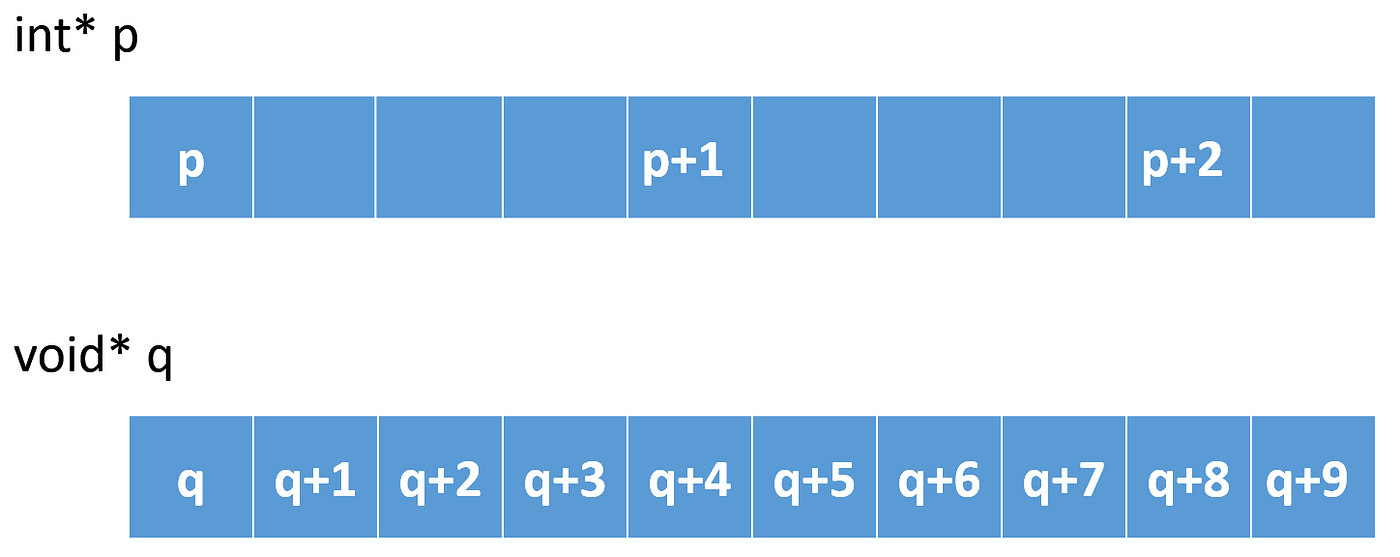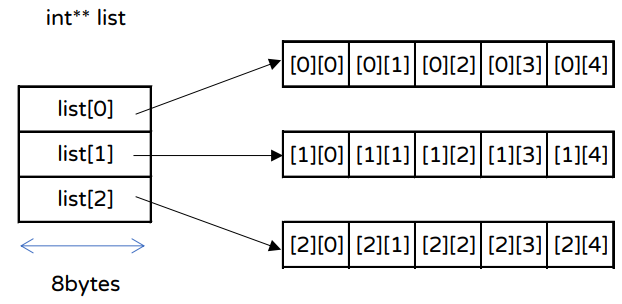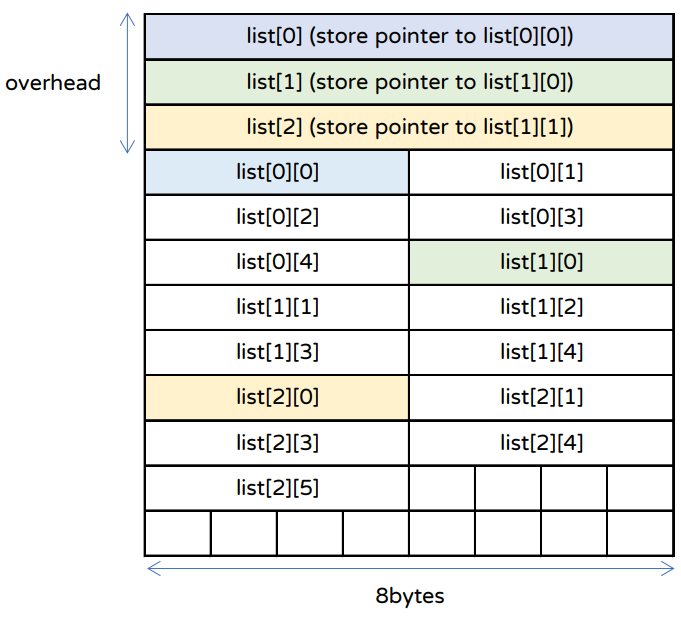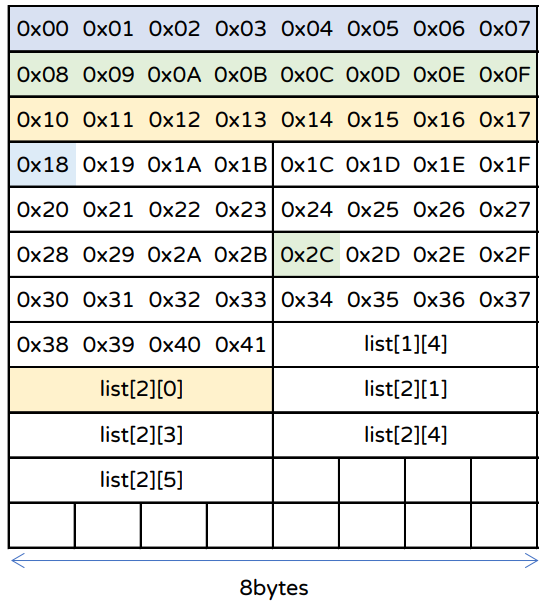# Memory layout

A program consists of the text segment and the data segment.

• The data segment is more complex than the text segment.Fig. 1 | Memory layout of a program.
1. text: contains executable instructions.
2. data, bss: global variable and static variable. The former stores initialized data, and the latter stores the uninitialized data.
3. stack: local variable in function. All local variables in a function appear in memory only when the function is called.
4. heap: provide a space for you to dynamic memory allocation. This space is managed by the OS. You can allocate space and release through OS. We usually use `malloc()` to allocate space without initialization, however, you can use `calloc()` to allocate zero-filled space.

# 1D Array

Before introducing how to use malloc(), we need to review a basic array in C. In fact, an array is a fixed block of memories. For example, we can declare an int array {10, 20, 30} before runtime.

`sizeof(int): 4list: 000000000061FE14list: 000000000061FE18list: 000000000061FE1C`Fig. 2 | Offset of a pointer.

# 2D Array

How about a 2D array?

`sizeof(int): 4list: 000000000061FE00 list:000000000061FE04list: 000000000061FE08 list:000000000061FE0Clist: 000000000061FE10 list:000000000061FE14`
`&list = *(list+2)+1list        = 0x61FE00list+2      = 0x61FE00+0x10 = 0x61FE10 (base+2*sizeof(int)*2)*(list+2)+1 = 0x61FE10+0x04 = 0x61FE14 (base+1*sizeof(int))`

# Malloc() & Free()

It is common that we cannot declare an array in bss, data, or stack segment without knowing the length of the list before runtime. Nevertheless, we can use a system call to request our operating system (OS) to give us a contiguous space in the heap segment at runtime.

1. If there is not sufficient space, malloc() returns NULL. Thus, you must check that the pointer that malloc() returned is non-null.
2. free(p) deallocates memories by given the first pointer of contiguous memory.

# void*

`void*` is a type that can store any pointer. Well, why do we need `int*`, `char*` , and other pointer types? Wanting to fetch the value to which the pointer points, we do not know how many bytes we should fetch, for example, `int` needs to read 4 bytes while `char` needs to read only 1 byte.

`int* p = (int*)malloc(sizeof(int));   // okint* p = malloc(sizeof(int));         // ok and concise`
`int num = 10;void* p = malloc(sizeof(int));*p = 10; // ERROR!!`

# Dynamically allocate the 1D array

1D array is a contiguous memory. For example, if we want to make an integer list that has 5 elements, we can allocate 5*sizeof(int) spaces.

# Dynamically allocate the 2D array

How to allocate a 2D array with 3 rows and 5 columns?Fig. 3 | Assume that sizeof(int*) = 8 and sizeof(int) = 4 in x86_64 system.

# Dynamically allocate the contiguous 2D array

The previous section needs to call malloc() 4 times when allocating and needs to call free() 4 times when deallocating. Can we just allocate by calling single malloc() and deallocate by calling single free() in order to improve performance?Fig.4(a) | Cotiguous allocate a 2D array where list list list stores int* value. list[m][n] stores int value. Assume that sizeof(int*) = 8 and sizeof(int) = 4 in x86_64 system.
1. list = *list+1 = 0x2C (type: int*)
2. jump to `*(list+1)+3` = `0x2C+3` where 3 is 3*sizeof(int) => 12bytes
i.e., fetch 0x2C+0x0C = 0x38
0x38 stores the value of integer.
`&list = *(list+1)+3list        = 0x00list+1      = 0x00+0x08 = 0x08 (base+1*sizeof(int))*(list+1)   = 0x2C*(list+1)+3 = 0x2C+0x0C = 0x38`Fig. 4(b) | Show the address of spaces in 3(a)

# Conclusion

1. The type of variable affects the offset of a pointer.
2. malloc() and free() are symmetric. You can only call free() as many times as you call malloc(). In addition, you can only free() the space which is allocated at runtime. i.e., you can NOT free the space in the bss segment, data segment, or stack segment.
3. malloc() is a kind of system call so you should call them as little as possible.
4. `void*` is just a type that can store a pointer. If you want to fetch the value pointed to by a `void*`, please convert the type first.
5. See full code at: https://github.com/liao2000/Medium/tree/main/how-to-use-malloc-and-free-in-C

--

--